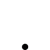# Electric charges part 1 longitudinal wave propagation

• 828 Views
• Last Post 30 January 2023
Vidura posted this 21 May 2022

This thread is a copy from the proboard forum. As the topic is important for understanding the principals of propagation of electric energy I decided to publish again in the new site. Eventually some details will be corrected by the way.

This thread will be dedicated to longitudinal waves and the related phenomenon's of physics. As an introduction I would like to remind you that the human brain has two hemispheres, each one dedicated to a specific kind of processing information. Nowadays the term knowledge is uniformly associated with the accumulation of information, and it's processing with a kind of binary logic. This capabilities are mostly located in the left hemisphere of the brain, in contrast the capacity to understand by the way of analogies , images and intuition are domains of the right hemisphere of the brain. There is certainly a insane unbalance of this capabilities in the present time, as from early stages of the educational system there is paid attention almost exclusive to the "left hemisphere intelligence", while the other side is left to its own, without any training in most cases. In order to develop the whole potential of our intelligence-knowledge, it is necessary to train both sides of our brain with their associated capabilities, as they are a conjugate pair. This will require some practice and time, but finally it will enable us to gain an integral understanding of the phenomenon's of the physical world and beyond, and transcend the limited "binary logic way of thinking" . This is the reason why we will use analogies of the properties of sound-waves in this topic, as it will help us to get a better visual and intuitive understanding of the electrodynamic equivalents.
First we will have a look at the properties of a sound-wave inside a tube, and analyse the processes in detail.
We will be discussing only the simplest form of waves (called linear waves). Most sound waves behave as linear waves since they produce pressure fluctuations in air that are very small compared to the atmospheric pressure. This will be sufficient for the beginning, as it represents quite good the basic behaviour of our analogy, which will be an electric conductor(long line).
Waves transfer energy without transferring matter.
Let us consider air particles set in motion by a vibrating piston. We can see that the particles (the black dots in the animation below; three of these have been coloured red for illustrative purposes) move back and forth about their equilibrium position, thus creating alternating zones of compression and rarefaction. In the rarefied region, the pressure is less than the normal undisturbed atmospheric pressure, denoted Patm, and in the compressed region, the pressure is greater than the normal undisturbed atmospheric pressure, as shown in the animation below.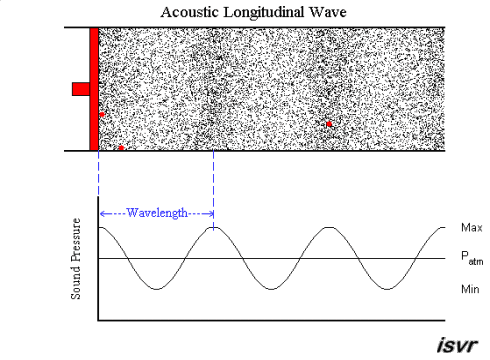As you can see, it is the disturbance which travels, not the individual particles (if in doubt fix your eye on one of the red particles). In sound waves, also known as acoustic waves, the local oscillations always move in the same direction as the wave. Waves like this are called longitudinal waves.
The velocity of a wave in a given medium (air, water, etc) is fixed and is related to the physical characteristics (temperature, density, etc.) of the medium.
But the frequency and thus the wavelength and the amplitude of the difference of pressure are dependent on the source, in our example from the movement of the piston.
For greater clarity, I selected key frames from this animation, which captured the individual stages of the red piston movement and the resulting deformation of the elastic air. Let's analyze them.

Below in the image, the piston (sound source) is on the left (at the bottom dead center) and starts moving to the right with some acceleration.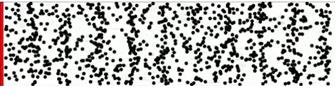When the piston moves to the right, air molecules condense in front of the piston, increasing the pressure in front of it (despite the tendency of the molecules to scatter in different directions).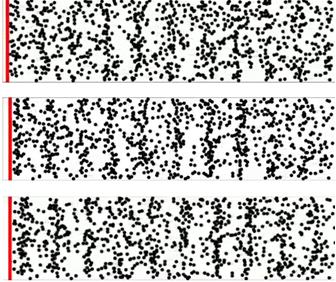In the upper image the piston continues its movement, forming condensations of molecules in front of it.

In the middle frame the piston continues to move, but already with maximum speed, and the wave of compression of molecules (but not the molecules themselves) in front of the piston continues to grow.

In the lower frame the piston stopped at the top dead center, forming a maximum concentration of molecules in front of it, after which the longitudinal wave, under the action of inertia, continues to move independently along the air channel at a constant speed.

Vidura

Vidura posted this 21 May 2022

This thread is a copy from the proboard forum. As the topic is important for understanding the principals of propagation of electric energy I decided to publish again in the new site. Eventually some details will be corrected by the way.

This thread will be dedicated to longitudinal waves and the related phenomenon's of physics. As an introduction I would like to remind you that the human brain has two hemispheres, each one dedicated to a specific kind of processing information. Nowadays the term knowledge is uniformly associated with the accumulation of information, and it's processing with a kind of binary logic. This capabilities are mostly located in the left hemisphere of the brain, in contrast the capacity to understand by the way of analogies , images and intuition are domains of the right hemisphere of the brain. There is certainly a insane unbalance of this capabilities in the present time, as from early stages of the educational system there is paid attention almost exclusive to the "left hemisphere intelligence", while the other side is left to its own, without any training in most cases. In order to develop the whole potential of our intelligence-knowledge, it is necessary to train both sides of our brain with their associated capabilities, as they are a conjugate pair. This will require some practice and time, but finally it will enable us to gain an integral understanding of the phenomenon's of the physical world and beyond, and transcend the limited "binary logic way of thinking" . This is the reason why we will use analogies of the properties of sound-waves in this topic, as it will help us to get a better visual and intuitive understanding of the electrodynamic equivalents.
First we will have a look at the properties of a sound-wave inside a tube, and analyse the processes in detail.
We will be discussing only the simplest form of waves (called linear waves). Most sound waves behave as linear waves since they produce pressure fluctuations in air that are very small compared to the atmospheric pressure. This will be sufficient for the beginning, as it represents quite good the basic behaviour of our analogy, which will be an electric conductor(long line).
Waves transfer energy without transferring matter.
Let us consider air particles set in motion by a vibrating piston. We can see that the particles (the black dots in the animation below; three of these have been coloured red for illustrative purposes) move back and forth about their equilibrium position, thus creating alternating zones of compression and rarefaction. In the rarefied region, the pressure is less than the normal undisturbed atmospheric pressure, denoted Patm, and in the compressed region, the pressure is greater than the normal undisturbed atmospheric pressure, as shown in the animation below.As you can see, it is the disturbance which travels, not the individual particles (if in doubt fix your eye on one of the red particles). In sound waves, also known as acoustic waves, the local oscillations always move in the same direction as the wave. Waves like this are called longitudinal waves.
The velocity of a wave in a given medium (air, water, etc) is fixed and is related to the physical characteristics (temperature, density, etc.) of the medium.
But the frequency and thus the wavelength and the amplitude of the difference of pressure are dependent on the source, in our example from the movement of the piston.
For greater clarity, I selected key frames from this animation, which captured the individual stages of the red piston movement and the resulting deformation of the elastic air. Let's analyze them.

Below in the image, the piston (sound source) is on the left (at the bottom dead center) and starts moving to the right with some acceleration.When the piston moves to the right, air molecules condense in front of the piston, increasing the pressure in front of it (despite the tendency of the molecules to scatter in different directions).In the upper image the piston continues its movement, forming condensations of molecules in front of it.

In the middle frame the piston continues to move, but already with maximum speed, and the wave of compression of molecules (but not the molecules themselves) in front of the piston continues to grow.

In the lower frame the piston stopped at the top dead center, forming a maximum concentration of molecules in front of it, after which the longitudinal wave, under the action of inertia, continues to move independently along the air channel at a constant speed.

Vidura

Vidura posted this 21 May 2022

Let's see the reverse movement now: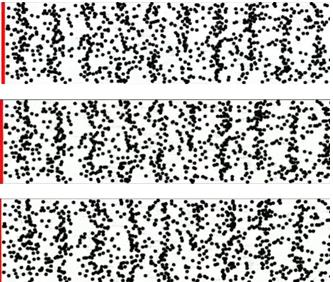In the top frame we see the beginning of the piston movement in the opposite direction - to the left. In this case, the reverse movement of the piston does not affect the movement of the longitudinal wave, and in front of the piston, the pressure in the air medium changes from normal to vacuum. Thus, oscillatory movements are generated in the air

in the middle frame it is clearly visible that the piston continues to move to the left side with maximum speed, but the deformation waves continue to move to the right side by inertia and managed to move away from the piston at a noticeable distance.

In the last frame the piston stopped at the bottom dead centre, forming a rarefaction zone in the air in front of it.

And so, three independent physical processes are clearly visible in the animation:

1) reciprocating oscillatory movements of the piston, creating longitudinal waves of variation of the density of molecules in the air channel in the form of compression and rarefaction, which do not affect the propagation speed of longitudinal waves;

2) a longitudinal wave in the air, propagating at a constant speed (about 340 m / s) and independent of the frequency of oscillations of the piston and their amplitude;

In the ideal case, if the molecules would not have chaotic thermal motions, then the longitudinal waves would have a coherent character.

3) molecules making reciprocating movements, like harmonic oscillations of a pendulum, which do not affect the speed of propagation of longitudinal waves in the air channel;
for each air molecule in reciprocating movements there are two points , beyond which they do not go out and, and in between them, remain motionless relative to the conductor. Moreover, the behaviour of molecules does not affect the propagation velocity of longitudinal waves, which is mainly a function of the distance between air molecules(density).
Let's transfer the data of these observations by analogy to electric charges in a conductor.

1. The action of a source of variable EMF in a conductor (similar to the action of a piston in an air channel), which creates in the conductor longitudinal waves of condensation and rarefaction of electric charges moving along the conductor ( always away from the source of the EMF) at a constant speed.

2. The wave propagates along the conductor (without insulation) at a constant speed of about 300,000 km / s which does not depend on the frequency of EMF oscillations and its amplitude;

3. Electric charges (like air molecules) will perform reciprocating movements in a conductor, like harmonic oscillations of a pendulum;

4. The points at which the oscillation of each charge stops will always remain in one place relative to the conductor, while the amplitude depends on the amplitude of the alternating EMF of the generator, but does not depend on its frequency.

From experience, we reliably know that voltage (and this is the phenomenon of compression-rarefaction of charges) and current (and this is the phenomenon of acceleration-deceleration during reciprocating movements of charges) are different phenomena, since in a circuit with a variable EMF they may not coincide in phase, which means that they are not immediately linked to each other.

Now we will look at some more general properties of longitudinal acoustic waves, if you can take a few minutes to watch the animations in detail, It might be helpful to imagine mentally the analogue behaviour of electric waves and charges in a conductor.
The animation below shows two acoustic longitudinal waves with two different frequencies but travelling with the same velocity. It can be seen that the wavelength is halved when the frequency is doubled: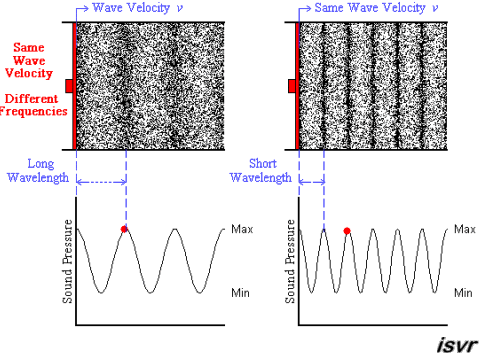The next animation shows two acoustic longitudinal waves with the same frequency but travelling with two different velocities. You can see that the wavelength is halved when the velocity is halved.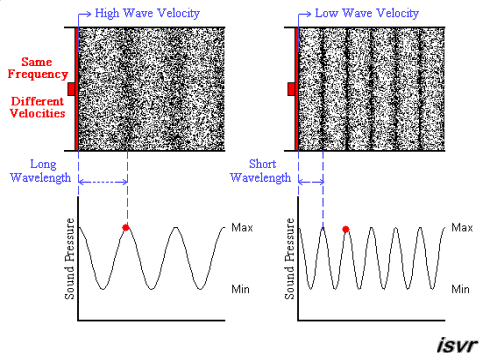The next topic is a very important one, as there are a whole lot of possible applications, not only in acoustics, but maybe still more in electric engineering:

The nature of standing waves

As practical guide it has to be remarked, that we only in very rare occasions deal with pure standing or travelling waves, in most situations both exist simultaneously in various degrees, likely one of both manifestations predominating.

Standing waves may be created from two waves (with equal frequency, amplitude and wavelength) travelling in opposite directions. Using superposition, the resultant wave is the sum of the two waves. The animation below shows that the net result alternates between zero (atmosferic average pressure) and maximum value of compression or rarefaccion.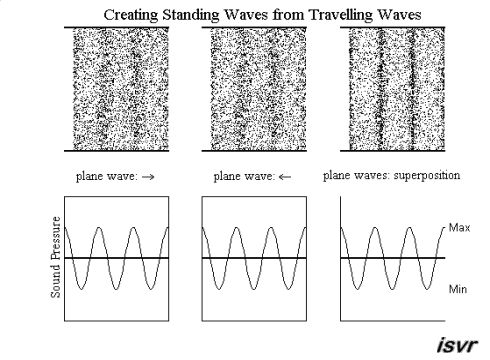For clarity of the definitions of travelling and standing waves I would like to focus your attention on the following aspect. It will be well understood, that in order to create a pure standing wave it is necessary to have two identical travelling waves in opposite directions. Then consider that one of these waves have a small difference in frequency from the other, for example 1Hz. As result we would see due to superposition a slowly “travelling” wave. But attention, the actual travelling waves are two, like in the former example.
Now let us continue to make our analogies with the electrical transmission line. It is clear, that there will by some differences, as we are dealing with charges, which are interacting with the environment causing many known and also still unknown physical effects. But for the basic behaviour of the wave propagation the comparison is well suited. In the case of the charges, we know that those of the same sign are repelling each other, due to the Coulomb forces. This fact makes them react to compression very similar as a gas, only that instead of elastic collisions the repelling force will transfer their kinetic energy to the adjacent charges.
We will in the following comparisons assume that positive charges are in a fixed position in the crystal lattice of the conductor, and negative charges (valence electrons) relatively free to drift across it. Thus, a region with compression of negative charges will have a negative sign, and a region of their rarefaction a positive sign.
If the length of the conductor with alternating current is of sufficient length, then we can register a charge density wave moving along the conductor with an electrometer or a voltmeter. If in some section of the conductor we observe a high electric potential (at the hot end of the coil), then by indirect signs we can conclude that in this place there is a concentration of charges of one sign or another. What else can explain the local increase in the electric potential? Indeed, without electric charges, there can be neither the potentials themselves, nor their difference. Consequently, the greater the concentration of charges of the same sign, observed in a certain place, the greater must be the electric potential created by these charges, and vice versa.

In order for us to find out in which part of the conductor there is a compression, rarefaction or equilibrium of the density of electric charges, we need an electrometer or voltmeter. But if we need to find out in which part of the conductor, for example, the deceleration of the movement of electric charges begins, then neither a voltmeter nor an Amperemeter that registers the uniform movement of charges will help us, we would need a radiometer. Radio waves, radiation of (electrically neutral) photons in the radio range, all these are different names for the same effect that occurs when the sign of the acceleration of the movement of charges changes. This will be discussed in more detail later.
Let us consider theoretically an example of registering an uneven potential along the length of conductors in an alternating voltage network of 50 Hz. The term variable means that:
1) voltage at the ends of the network changes twice for a period of 0.02 sec. its sign;

2) the current changes its direction twice during the period;

3) the current changes the sign of the acceleration of charges four times during the period;

4) in the phase of deceleration of charges, the conductor always emits energy in the form of photons;

In a single-phase electrical network of alternating voltage with a frequency of 50 Hz or 60Hz, the wavelength λ is very large and reaches - from 5,000 km to 6,000 km (depending on the materials used for conductors and insulators). Therefore, it is possible to register a change in potential in a section of the circuit, but on condition that its wavelength is at least ¼ λ, that is, it will be in the range from 1,250 to 1,500 km. You will agree that in such a line it is very difficult to register a local change in the electric potential or the peak of the compression or rarefaction of charges due to the long wavelength.

Vidura

Vidura posted this 21 May 2022

The fact that the voltage in the circuit is alternating, we can measure if we connect a voltmeter to the ends of the circuit to measure alternating voltage.

We can proof that an alternating current flows in the circuit if we install a shunt or a current transformer and indirectly measure the current in the circuit.

Rarely anyone had asked the question if the network emits a radiation, and in general we never think about it, so we don't even have specialized devices for this (except for some modern electronic probes). On the other hand, antenna specialists are familiar with the radiation of radio waves (or photons of the radio range; although they call radio waves and other emissions of photons erroneously electromagnetic waves). It is clear, that these photons have a much shorter wavelength than the one calculated by the varying EMF fed to the antenna system (primary modulation, longwave; shortwave and other ranges) since the frequencies of the photons themselves lie in the range of x-rays or gamma radiation, which can be calculated with the formula ΔE = ν · h, where ν is the frequency, h is Planck's constant.
Let's try to understand these questions again with the help of visual drawings and start from the very beginning - with the action of the variable EMF and the longitudinal waves that arise in this case.

We will assume that alternating voltage is moving at a constant speed (but not electric charges) as longitudinal waves, which are compression and rarefaction of the density of electric charges in a conductor. In this case, outside and inside the conductor, specific physical effects arise, which will be discussed in other threads.

The figure below shows the sections of the conductor, on which negative charges are compressed, coloured in blue, and the sections of rarefaction of negative charges coloured in red, and in the white sections, the density of electric charges is in relative equilibrium.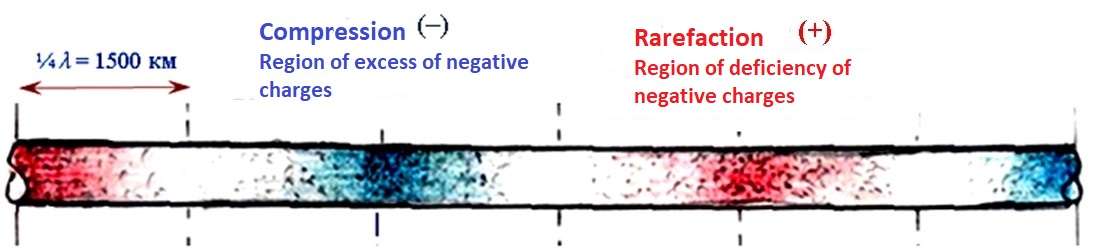This is an illustration of a longitudinal charge density wave in a conductor, which is completely analogous to a sound longitudinal wave propagating in a long air channel.

Under normal conditions, negative electric charges in a metal conductor are located near the nodes of the crystal lattice (which have a positive charge), while their density is the same throughout the entire conductor, and the conductor as a whole is electrically neutral.

If a source of constant EMF(DC source) is connected to such a conductor, then the electric charges in the conductor will begin to drift along the nodes of the crystal lattice at a constant speed(electron drift velocity), while the charge density along the entire conductor will also remain constant.

But if a source of variable EMF is connected to a conductor (in a closed circuit), then a longitudinal wave (deformation of the density of electric charges) will appear in the conductor, which is a movement along the conductor, but not of the electric charges themselves, but longitudinal waves of compression and rarefaction of charges. In this case, relative to the crystal lattice of the conductor, the charges themselves will actually remain in place, making only reciprocating movements along its length (within ½ the length of the longitudinal wave). For an external observer, such a movement of charges would appear like the oscillation of a pendulum, its movements are determined by the frequency and amplitude of the variable EMF source. The centre of its oscillations is motionless relative to the conductor!
Let us list again the processes which arise in the conductors:

1) there are moving waves of compression and rarefaction of the density of electric charges in the conductor, alternating along the entire conductor and located at a distance of ¼ wavelengths from each other.

2) these waves continue to move in the conductor "by inertia" at a constant speed in the direction from the generator, due to the exchange of momentum between the adjacent charges;

The term "by inertia" is taken in quotation marks, since in fact the charges continue to move due to the phenomenon of the EMF of self-induction in the conductor, which apparently looks like mechanical motion by inertia. So, these movements turn out to be completely analogous.

3) the resulting imbalance in the density of electric charges inside the conductor leads to a return movement, but not of waves, but charges themselves in the direction towards the source;

4) the return movement of charges restores the pressure in the zone of rarefaction of charges.

5) the movement forward and back of the charges occurs with the same speed and acceleration, the charges in the conductor remain in it in the same section, while making oscillatory movements along the conductor with an amplitude equal to ½ wavelength.

It is important to note that the movement "by inertia" of the compression-rarefaction waves from the EMF source along the conductor at a constant speed is primary, and the reaction in the form of the movement of charges in the opposite direction is secondary. This means that the voltage in the conductor is always primary and the current is secondary.

Let us consider in the illustrations the propagation of longitudinal waves of electric charge density in more detail (by analogy with the propagation of sound waves) in an open and closed long line:

1) the propagation of a longitudinal wave in air at a constant speed of about 340 m / s, which does not depend on the frequency of oscillations of the piston and their amplitude;

The EMF of a generator, like the movement of a piston in a cylinder, deforms the density of electric charges in different ways, we will call the density of charges in the conductor compression, normal and rarefaction of charges. Below is conventionally shown a linear chain of charges standing one after another, the momentum vector P of a moving charge and three states of charge density –Let's remember that the charges are repelled, therefore, when similar charges approach each other, the Coulomb force acting between them increases, which allows them to exchange momentum without collisions.

Shown below is a section of a two-wire long symmetric line (the dielectric is air) and the deformation of the charge density in it, the oscillation frequency of the generator EMF is 50 Hz, the wavelength λ in such a line is about 6000 km (1/4 λ = 1500 km). Let us remember that in air longitudinal waves in conductors propagate along the entire line at a velocity of about 300,000 km / sec, and this velocity is dependent on the dielectric surrounding the conductor , more precisely  on the distributed capacitance of the line. This can be measured, and for example for a PVC insulated wire laying on the ground, the velocity of propagation is 170000km/sec approximately. The most important parameters for the distributed capacitance are the surface and shape exposed to the second conductor or ground plane, the distance between them  and the dielectric constant.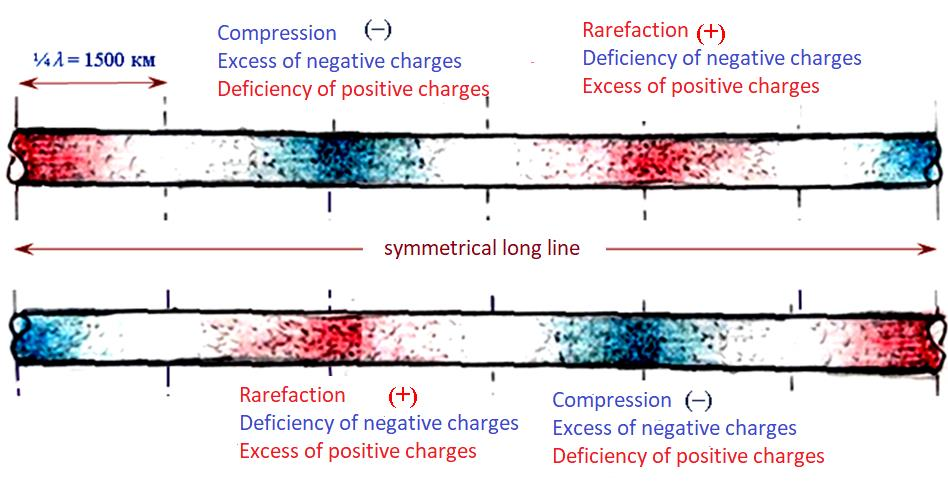The figure shows that in the sections of the circuit where the concentration of charges is indicated, there is a minus sign, where the discharge of charges is indicated, there is a plus sign. Indeed, a negative electric potential can arise only where negative charges are in excess, and a positive potential only where there are fewer negative charges, that is, where only immobile positive metal ions remain at the nodes of the crystal lattice.

Moreover, the direction of longitudinal waves in both conductors of a symmetrical line coincides in direction with the action of the EMF, as shown in the figure below.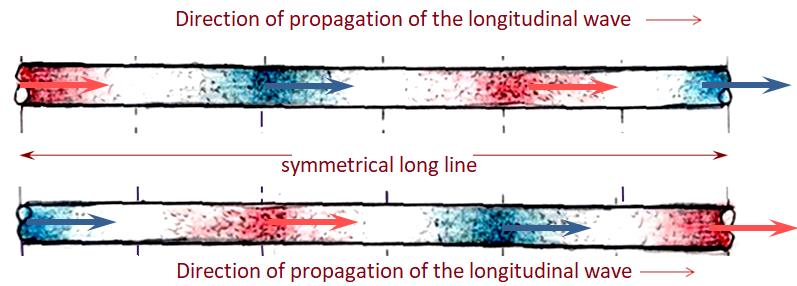This fact is the source of a phenomenon called phase displacement in the line between current and voltage. This will be discussed in more detail. Will  be continued.

Vidura

Vidura posted this 23 May 2022

If an air channel with propagating sound waves is blocked at some distance, then reflected waves will appear in it; As a result of the superposition of the incident and reflected waves, standing waves will appear in the channel. Let us consider in the illustrations in more detail the analogy of these processes in a long line. We have to note, that the analogy for a closed pipe would be an open-ended transmission line, which would reflect the longitudinal waves.

Let's start with the fact that the sections of long lines, on which the greatest acceleration of charges is observed, may not coincide with the sections, in which the greatest rarefaction of charges is observed. And, conversely, the sections of the line, in which the greatest deceleration of charges is observed, may not coincide with the sections of compression of charges. In conventional terms, current and voltage fluctuations in long lines are out of phase with each other. Why does this happen?
For comparison, the figures below once again show the relative position of the sinusoids of current (blue) and voltage (red) in open and closed lines. It should be noted that for more clarity, only incident waves are shown on the line sections and not their reflections.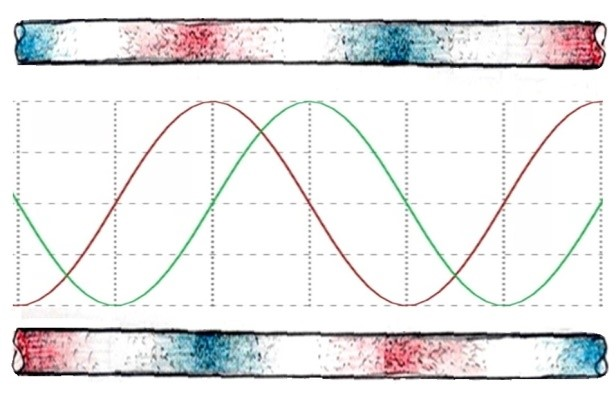An open line has a predominate capacitive nature, therefore, the sections of the line with the maximum velocity of movement of charges (current) are ahead of the time of compression or rarefaction of the charge density (voltage) in phase by 90 degrees, as shown in the figure above. In conventional terms, in an open line, the current phase is ahead of the voltage.  At the endpoint of an open-ended transmission line always an  antinode (maximum) of potential and an node(zero) of current appears.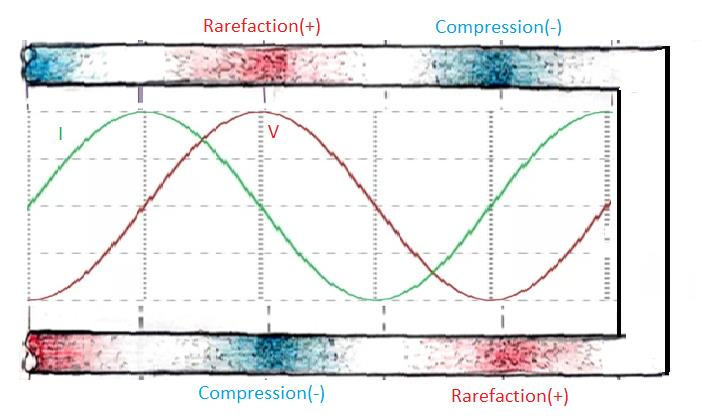A closed line has a predominate inductive nature, therefore, the sections of the line with the maximum velocity of movement of charges (current) lag in time from the sections of compression or rarefaction of the density of charges (voltage) in phase by 90 degrees. In conventional terms, in a closed line, the voltage phase is ahead of the current. At the endpoint of a closed (shorted) transmission line always an antinode (maximum) of current and an node(zero) of potential appears. We can assume that in this case the longitudinal wave from the upper line passes thru the endpoint to the lower line and vice versa, this is the reason for the different displacement of the phases.

The only case when there are no reflected waves in the line, and the current and voltage are exactly in phase, is when a matched resistive load is included in the line. This case is shown in the figure below, where the amplitudes of voltage (red sine wave) and current (blue sine wave) are presented with different amplitudes (for clarity).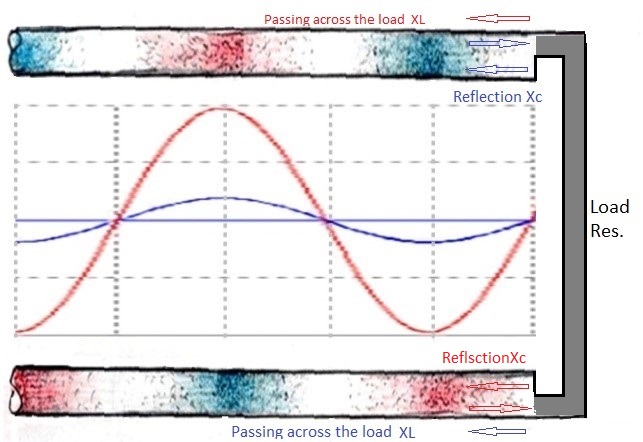In other cases, depending on the magnitude of the active load in the lines, there are always, but to a different extent, two waves are present and interact with each other. First the incident waves propagating away from the source of EMF, and second waves of the opposite direction, which can have two different origins: Reflected waves, if the line is prevailing capacitive, and waves that have passed across the load from the upper line conductor to the lower one and vice versa, if the inductive reactance of the line prevails. It is their interaction that we observe in the lines, and which causes the shifting of phases to one or another side by superposition.

In the context of the above-mentioned interaction of waves we can now understand the meaning of what happen, when a matched resistive load is connected to a symmetrical transmission line. Capacitive reactance means the waves are reflected at the endpoint(load) with the current wave 90° ahead of the voltage wave, inductive reactance means that the waves are passing trou the endpoint interchanging the lines, with the current wave 90° behind the voltage wave.Capacitive reactance(Xc) means the waves are reflected at the endpoint(load) with the current wave 90° ahead of the voltage wave, inductive reactance(XL) means that the waves are passing across the endpoint interchanging the lines, with the current wave 90° behind the voltage wave. A matching load means that both, capacitive reactance and inductive reactance are equal of opposite sign, and thus the returned waves(reflected and passing the load) are at 180° in anti-phase cancelling each other. No returned waves and thus no standing waves are observed in this case.
Now we will briefly touch the topic of resonance in this context also. It is important to know, that only when the wavelength and the total length of the line are such, that the returned waves at the EMF source are matching in sign and phase, we can observe the phenomenon of resonance, leading to positive feedback which can lead to exponential increments of the values. The resonance can occur for current and voltage waves, but only either one or the other, unless two EMF sources would be present on both ends of the line.
So, it is understandable and straightforward, that power transmission lines should never come into resonant conditions, as the effects would be devastating.

Vidura

Vidura posted this 23 May 2022

Now let’s look a bit closer at the analogy of the reciprocating movement of air molecules in the acoustic channel to the movement of electric charges. I recall that we do not have instruments for direct measurement of the movement of charges, only indirectly with the methods of shunt resistors, current transformers or hall effect detectors we can get information about their movements. In the following images we can see a pendulum as representation of the oscillations of the charges in an open-ended and in a closed line. The letter a means the acceleration with positive sign, or deceleration with negative sign, when the pendulum is at the centre position v max indicates the maximum velocity.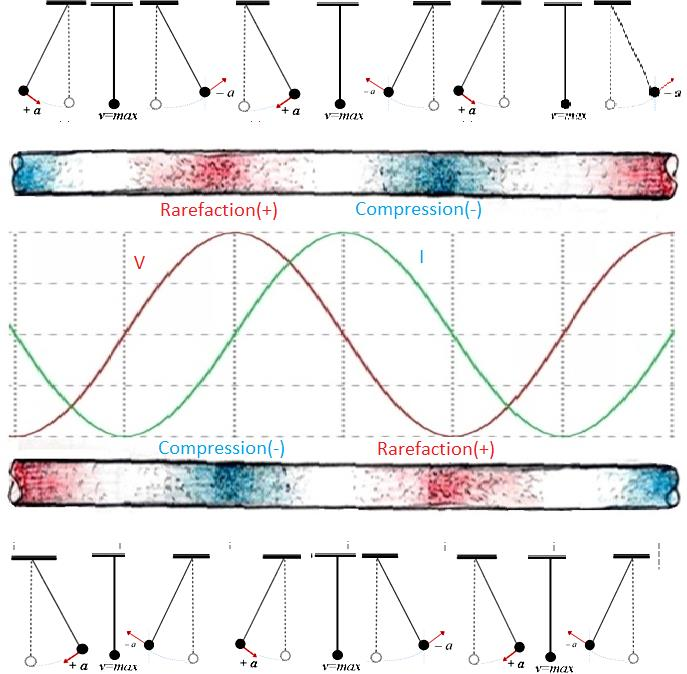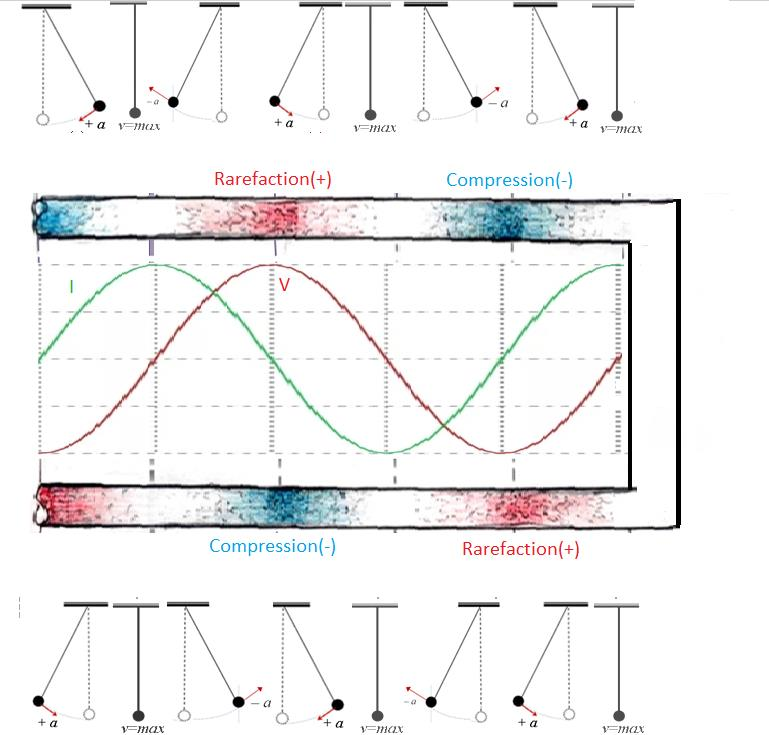The Images show that the pendulums in the upper line swing always in the opposite direction than in the lower line, maintaining thus the overall balance of the system.
From observing sound waves and comparing them with electricity, it became possible to draw an important conclusion that in a long line two different processes are present, relatively independent of each other - this is the movement of waves of compression and rarefaction of charge density and reciprocating motion charges- in electrical terms, are voltage and current.
The last thing we still have to focus on is the phenomenon when the movement of molecules is decelerating. In air, this phenomenon is not observed in the same way as in a long line, but with this effect it is possible to create refrigeration and heating installations, which can operate with the  energy of reciprocating movements of air molecules only. This becomes possible if standing or travelling waves are created in the air channel. We have already covered this in the thread “concepts of AU devices”.

In the figure above the regions indicated with a negative acceleration of charges(a-) are located in the second and in the fourth quadrant of the current sinusoid, or between the maximum and zero-crossing. It turns out that at the moments when the charges begin to decelerate, they begin to emit the energy received during the acceleration in the form of radiation of photons. Remember the effects described in the posts about the Franck-Hertz experiment. It turns out, that atoms can be exited by different means, for example thermal energy or applying an EMF. When the atom gets excited, it absorbs photons which are in a matching range of wavelengths from the always present background radiation. In this process electric charges(electrons) are accelerated to greater velocity (in the reciprocal oscillation process). When they begin to decelerate until the point of zero velocity, they will emit the same amount of energy by radiation of photons. We could assume that while the charges in a conductor are in movement at higher velocity, their average distance form the positive nuclei is greater, and when the velocity decreases, they will take positions closer the nuclei, returning to the ground state of orbitals when the velocity crosses thru zero.

Vidura

Vidura posted this 23 May 2022

What is a wave?

A wave is by definition a disturbance in a medium. Therefore we must assume the presence of a medium when we talk about a wave. In the case of water waves or soundwaves things are quite straightforward. But in the case of electrodynamic and magnetics it is not so simple, as we must deal with invisible forces and mediums.

What different kind of waves can be observed?

There are four types of waves differing in the pattern of propagation.

The first is the scalar wave, that means that  in a contiguous medium a wavefront expands  in a spherical shape from a point source in all directions.

The second type is the longitudinal wave, which propagates in a medium constrained in a conduit, and the deformation of the medium occurs in the direction of the propagation.

The third type is called transvers wave, which is defined by the deformation of the medium  orthogonal to the direction of propagation.

The fourth type of waves is the vortex , or torsion wave in solid state mediums.

As someone correctly acquainted, in most cases there are combinations of the different wave types in practice. For example a  longitudinal pressure wave in a hose goes along with a transverse wave of deformation of the sidewalls of the hose. In Electrodynamics a potential wave is associated with a wave of displacement, and the latter with a vortex movement or a torsion field manifesting effects known as magnetism.

Conventions and physical models:

We have to remember, that the examples in this topic are based on physical models, as for example Bohr's atom model, supposing that the "subatomic particles" are tiny spheres of some solid substance. But it is likely, and many results of latest scientific research supports this, that what we perceive as matter is more precisely something like standing waves at subatomic scale. I don't want to go deeper into this subject because my knowledge in theoretical physics  and mathematics is not complete enough, and also because there would be only a few who could follow.

The intention of the topic is to be a guide for an intuitive understanding about the behaviour of waves and their propagation, and  discuss considerations for the practical implementation in resonant circuits.

In the following posts I will show some tests of measurement of the wave propagation, which I think could be helpful for those who are building on replications.

In the context of this topic I would like to draw your attention to this video posted by fighter in the thread about Litz wire:

This fellow has a quite good understanding of transmission lines judging by the interpretations of his experiments. Regarding his comment about the function of photons in the wavepropagation I was quite surprised, as this is usually completely ignored or unaccepted in conventional physics. We will come back to this in another thread for looking closer to this theory.

Vidura

Vidura posted this 24 January 2023

Hi all, when I made measurement of resonance in a wire, I found that the the theories proposed by Ivor Catt( based on Heavysides concept) are going in the right direction. Here a video of my test results:

What is your opinion how the potential can appear on the second terminal of the wire , to which only the high impedance scope probe is connected, in 20ns? Regards Vidura

Vidura

realco posted this 25 January 2023

Hi Vidura.
A complex material for most of us I think, but a good analogy with sound propagation.
If we were to extrapolate a little... do you think it would be possible to give an example of a circuit, device, etc. which can be achieved? What do you think about the caduceus coil, for example. Would it fit in here?
In any case, it is one of the few articles that deal with this subject.

Shelfordella posted this 25 January 2023

"A little theory and calculation would have saved Edison 90 per cent of the labor." Nikola Tesla

Vidura posted this 26 January 2023

Here I will  add some scope shots of the actual resonance test of the wire: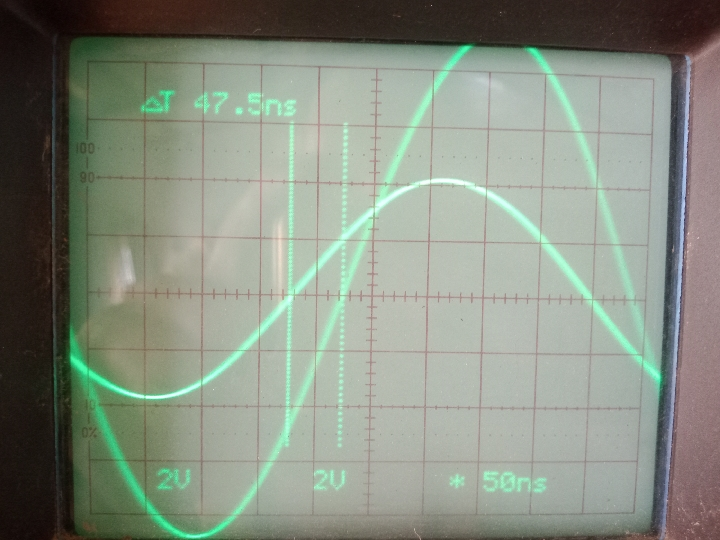Ween the wire comes in resonance, the phase delay is according to the speed of light for the lenght of the cable.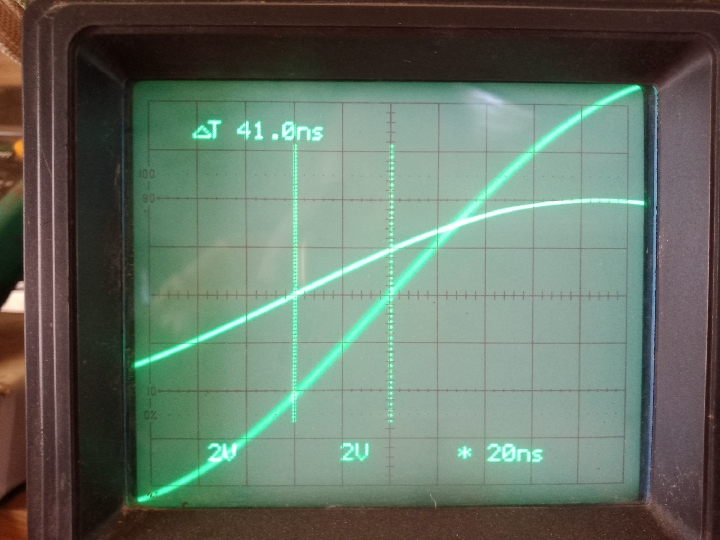BUT, when a pulse with a low duty cycle is  inyected , the potential appears after 20ns on the other end of the same wire (aprox. 15 meters), it is independent of the frequency of the squarepulse. It also do not change if the scope and SG is grounded or floating.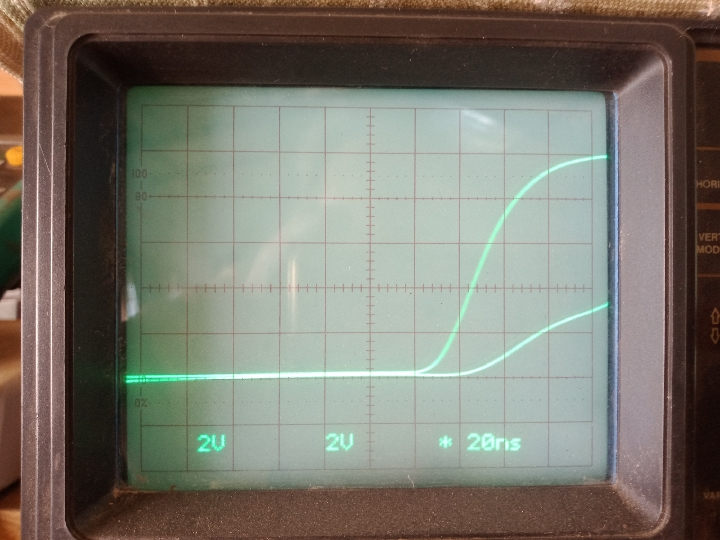Remember that on one end of the wire there is the SG+plus and CH1 conected, and on the other end CH2, no ground connection on the wire. The SG-minus and GND of the scope probe are joined together.

According to the theory adopted by Ivor Catt a energy wave propagates away from the source along the wire, and two signals of oposite(electric) polarity and half of the applied potential travels across space or the medium along the wire , in this case along both wire ends , and when they meet at the centrepoint(or a load in a two wire line) the electric potential appears between the two terminals.

Vidura

Vidura posted this 27 January 2023

Quoting:Shelfordella